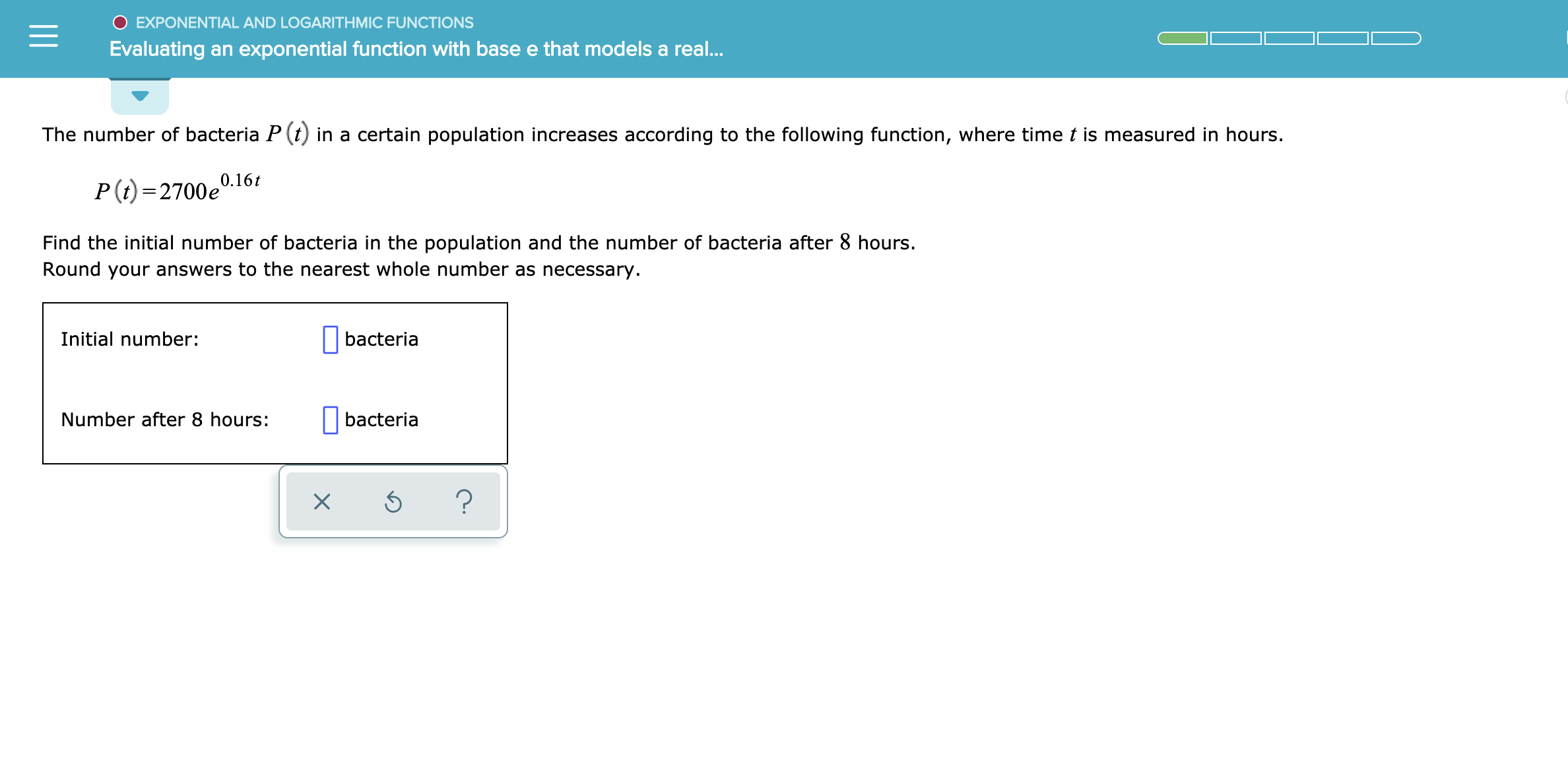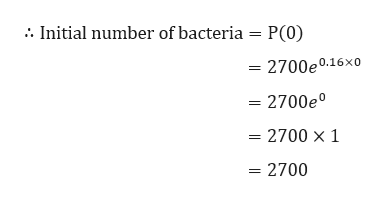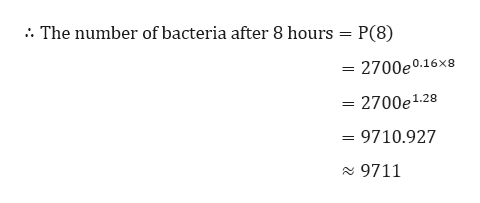# O EXPONENTIAL AND LOGARITHMIC FUNCTIONSEvaluating an exponential function with base e that models a rea...The number of bacteria P (tin a certain population increases according to the following function, where time t is measured in hours.0.16tP (i) 2700eFind the initial number of bacteria in the population and the number of bacteria after 8 hours.Round your answers to the nearest whole number as necessaryInitial number:bacteriaNumber after 8 hours:bacteria?X

Question

See attachmenthelp_outlineImage TranscriptioncloseO EXPONENTIAL AND LOGARITHMIC FUNCTIONS Evaluating an exponential function with base e that models a rea... The number of bacteria P (t in a certain population increases according to the following function, where time t is measured in hours. 0.16t P (i) 2700e Find the initial number of bacteria in the population and the number of bacteria after 8 hours. Round your answers to the nearest whole number as necessary Initial number: bacteria Number after 8 hours: bacteria ? X fullscreen
check_circle

Step 1

Given,

Step 2

Now to find the initial number of bacteria populations put t = 0 in the above equation, we gethelp_outlineImage TranscriptioncloseInitial number of bacteria = P(0) = 2700e0.16x0 = 2700e° = 2700 x 1 = 2700 fullscreen
Step 3

To find the number of bacteria after 8 hours p...help_outlineImage Transcriptionclose. The number of bacteria after 8 hours = P(8) = 2700e0.16X8 2700e1.2 = 9710.927 29711 fullscreen

### Want to see the full answer?

See Solution

#### Want to see this answer and more?

Solutions are written by subject experts who are available 24/7. Questions are typically answered within 1 hour.*

See Solution
*Response times may vary by subject and question.
Tagged in

### Functions